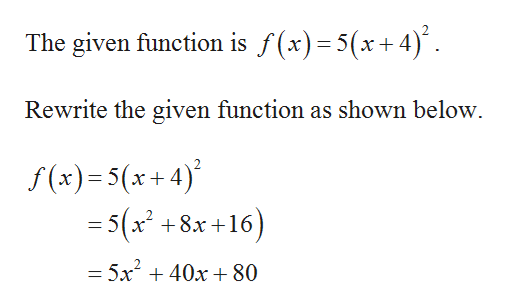# Is the function f(x)=5(x+4)2  a power function? If so, write the function in the form f(x)=k⁢xp. If it is not a power function, enter NA for k and p. Otherwise enter the exact answers.k= p=

Question
1 views

Is the function f(x)=5(x+4)2  a power function? If so, write the function in the form f(x)=k⁢xp.

If it is not a power function, enter NA for k and p. Otherwise enter the exact answers.

 k= p=
check_circle

Step 1help_outlineImage Transcriptionclose= X Rewrite the given function as shown below f (x)=5(x+4) =5(x- x28x16 5x 40x80 fullscreen

### Want to see the full answer?

See Solution

#### Want to see this answer and more?

Solutions are written by subject experts who are available 24/7. Questions are typically answered within 1 hour.*

See Solution
*Response times may vary by subject and question.
Tagged in

### Other